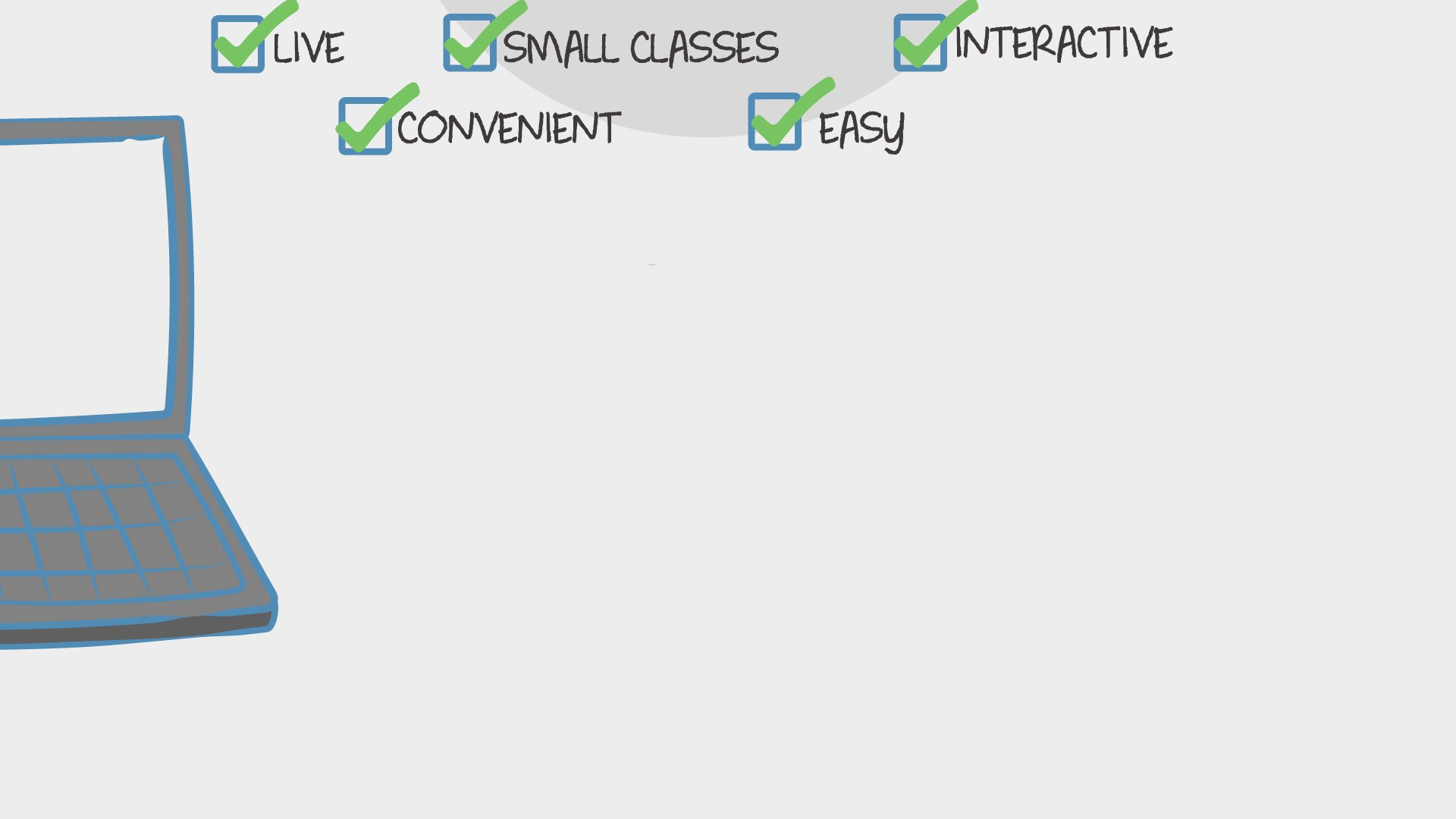Math

## Pre-Algebra 8th Grade - Part 2

Students will learn and master introductory algebra concepts that will prepare them for Algebra 1.
66 total reviews for this teacher
2 reviews for this class
Completed by 8 learners
There are no upcoming classes.per class

over 18 weeks

year olds

#### 3-6

learners per class
per learner - per class

### How does a “Multi-Day” course work?

Meets multiple times at scheduled times
Live video chats, recorded and monitored for safety and quality
Discussions via classroom forum and private messages with the teacher
Great for engaging projects and interacting with diverse classmates from other states and countries### How Outschool Works### There are no open spots for this class.

You can request another time or scroll down to find more classes like this.

## Description

#### Class Experience

```Students learn extensively from and by examples, and through problem discussions. A variety of instructional methods are used to enhance students' problem-solving abilities. Students tackle many complicated problems using inductive and deductive reasoning approaches.  Students will be prepared to:
- understand that solutions to systems of two linear equations and inequalities corresponds to the intersection of their graphs.
- solve systems of two linear equations and inequalities algebraically, and graphically.

-construct and interpret scatterplots to investigate patterns between two quantities
-identify categorical data by displaying frequencies and relative frequencies in a two-way table. Construct and interpret a two-way table summarizing data on two categorical variables collected from the same subjects.
-Use the equation of a linear model to solve problems in the context of bivariate measurement data, interpreting the slope and intercept.

-Verify experimentally the properties of rotations, reflections, and translations.
-Understand that a two-dimensional figure is congruent to another if the second can be obtained from the first by a sequence of rotations,
reflections, and translations.
-Describe the effect of dilations, translations, rotations, and reflections on two-dimensional figures using coordinates.
- given two similar two-dimensional figures, describe a sequence that exhibits the similarity between them.
-Use informal arguments to establish facts about the angle sum and exterior angle of triangles, about the angles created when parallel lines are cut by a transversal, and the angle-angle criterion for similarity of triangles.

-Apply the Pythagorean Theorem to determine unknown side lengths in right triangles in real-world and mathematical problems in two and
three dimensions.
-Apply the Pythagorean Theorem to find the distance between two points in a coordinate system.
-Know the formulas for the volumes of cones, cylinders, and spheres and use them to solve real-world and mathematical problems.
```
`I love teaching mathematics to students.  I work hard to learn how my students learn and make adjustments accordingly. I am highly qualified to teach 5th grade to 12th grade mathematics.`
`The learner will be assigned weekly lesson assignments and exit quizzes. They will be graded and returned to the learner.  Lesson assignments will have a weighted grade of 10% of overall grade.  Lesson Quizzes will have a weighted grade of 30% of overall grade.`
```.css-jzlc08{margin-top:0.5em;margin-bottom:0.5em;}  1 file available upon enrollmentI will supply curriculum material by posting lessons weekly in OUTSCHOOL.  The student will need the following:
Materials: Each student must bring the following to class meetings weekly:
1. Pen and pencil (including colored pencils)
2. Texas Instrument Calculator (Ti-83 or Higher) or on-line graphing calculator DESMOS
3. Folder (preferably a 3-ring binder with dividers) to hold class work and assessments
4. Graph Paper
5. Highlighters for notes```
`In addition to the Outschool classroom, this class uses:DESMOS Graphing CalculatorOn-Line PracticeReference TextbookIXL Learning`
```Requirements:  Students should expect to have homework almost every day/night in this class.  It is strongly recommended that students set aside at least 20 minutes per day for doing homework.  Homework will be graded for understanding each week. Homework is 10% of overall grade.

Quizzes and tests make up the majority of a students’ course grade.  But remember, if you aren’t practicing the math we are learning by doing your homework and class work on a regular basis,  your scores on these quizzes/tests will more than likely be lower than they would be if you did do your homework every day.   Quizzes are 25% and Tests are 60%of overall grade.

Other possible grade includes attendance and participation.  Attendance/participation is worth 5% of overall grade.
A      90-100%      Exceeds Expectations; Independent learner; superior work; all work completed on
time at an exceptional level of quality and effort;
B      80-89%      Meets Expectations; Semi-independent learner; above average work; all work
completed on time; did quality work with a few flaws;
C      70-79%      Passing but does not meet some standards; Dependent learner; average work; most
completed on time showing average effort;
D     60-69%      Passing but only meets minimum standards; Dependent learner; average work; most
completed late showing below average effort;
F      <60%         Failing, does not meet minimum standards; Failure; does not meet standards or expectations.
```
`1 hour per week in class, and an estimated 2 - 4 hours per week outside of class.`

## Teacher

```I am a highly qualified mathematics and chemistry teacher.  I taught 10 years in the middle school and 10 years at the high school.  I love teaching and believe in innovative ways to keep students motivated and engaged.